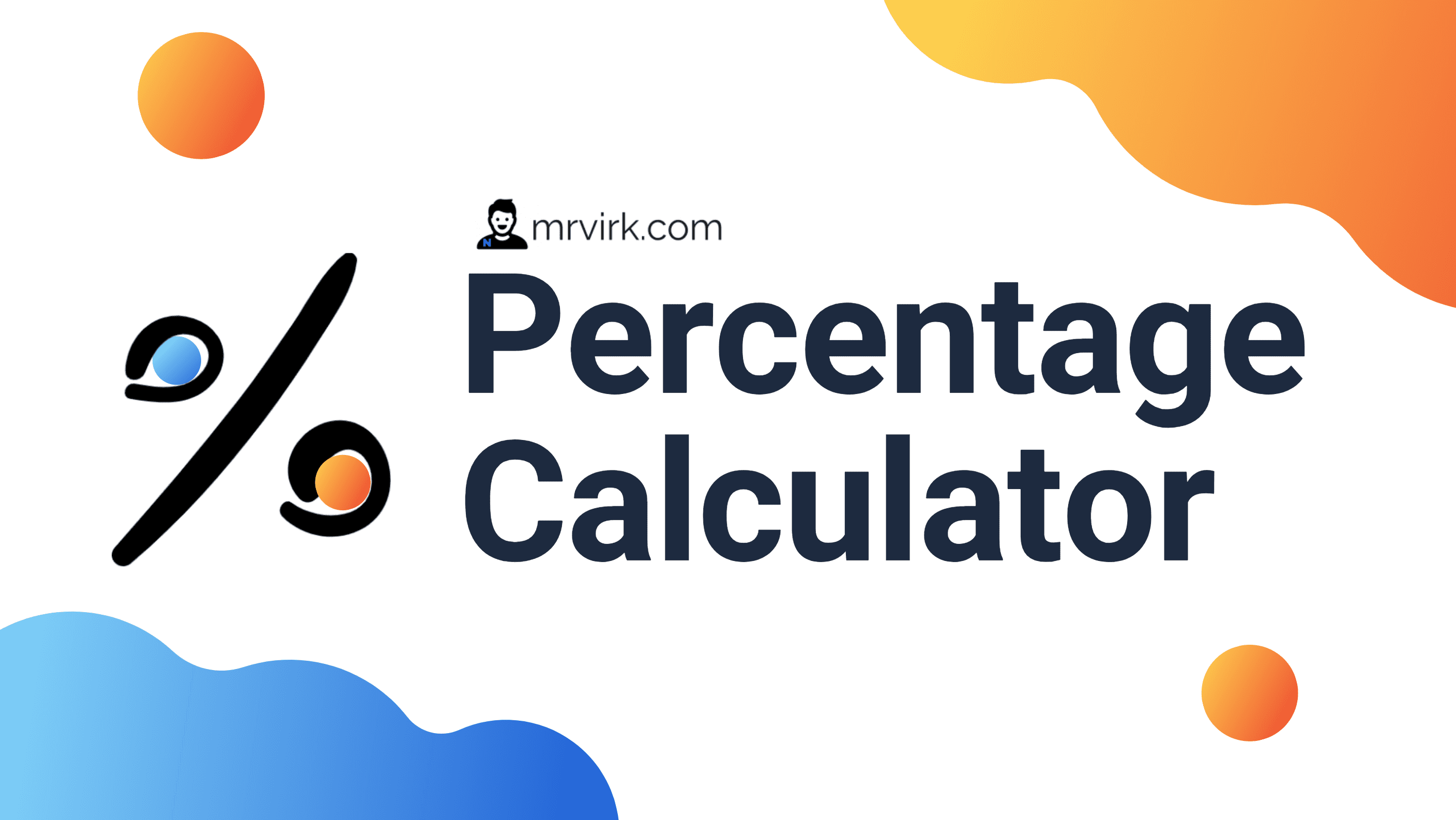# How to Calculate Percentage - Formula and ExplanationYou will learn to Calculate Percentage, this page explains - What is Percentage ? Explanation and Formula.

## What is Percentage ?

A Percentage is a number or ratio expressed as a fraction of 100. It is often denoted using the percent sign, "%"

## Percentage Formula

Find What % is x of y ?
Percentage can be calculated by dividing the new number (x) with principal number/amount (y) and mutiplying with 100. So, Percentage Formula, p = (x/y)*100

Examples:
p = (x ÷ y) * 100 = (10 ÷ 100) * 100 = 10%
p = (x ÷ y) * 100 = (25.5 ÷ 100) * 100 = 25.5%
p = (x ÷ y) * 100 = (100 ÷ 400) * 100 = 25%

## Real Time Formula Explanation

Now, Try the more comprehensive Calculator that also explains the result and the formula used for Calculating Percentages for your inputs in the calculator

Go back to basic calculator

x is what percent of y ?

is what percent of ?

Result
Formula

What is _ % of y ?

What is % of =

Result
Formula

Profit/Loss Percentage Calculator

Starting amount is and new amount is
The change in % is =

Result
Formula
Go back to basic calculator